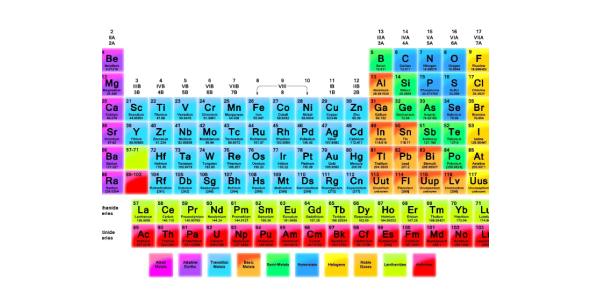# The Periodic Table Of Elements Quiz

16 Questions | Total Attempts: 46SettingsDo you know about all the elements that are in the periodic table and their recurring properties? Well, if you are well familiar with the periodic table, then let's see if you can answer all these questions or not. All the best!

• 1.
What is the relationship between atomic number and atomic radius as you go down each group in the periodic table?
• 2.
What is the atomic number of this element?
• 3.
. What is the name of this element?
• 4.
What is the atomic mass number of this element?
• 5.
How many valence electrons does this atom have?
• 6.
How many energy levels does this element have?
• 7.
What group does this element belong to?
• 8.
• 9.
The zigzag line on the periodic table divides
• A.

Alkali metals and transition metals

• B.

Metals and nonmetals

• C.

Semimetals and transition metals

• D.

Inert gases and halogens

• 10.
The smallest particle into which an element can be divided and still be the same substance is a (n)
• A.

Electron

• B.

Proton

• C.

Atom

• D.

Molecule

• 11.
Which group contains elements whose atoms have 4 electrons in their outer level?
• A.

Group 3

• B.

Group 14

• C.

C.Group 4

• D.

Group 15

• 12.
4. The first periodic table arranged elements in order of
• A.

Increasing atomic mass

• B.

Decreasing atomic mass.

• C.

Increasing atomic number.

• D.

All above

• 13.
How is the Periodic Table of the Elements organized?
• 14.
According to the periodic table, which of the following elements is the most chemically reactive nonmetal?
• A.

Lithium

• B.

Oxygen

• C.

Fluorine

• D.

Phosphorus

• 15.
Why is the periodic table called the periodic table? (Think about your graphing activity.)
• A.

True

• B.

False

Related TopicsBack to top